# Functions f A B Lecture 12 Functions function

• Slides: 13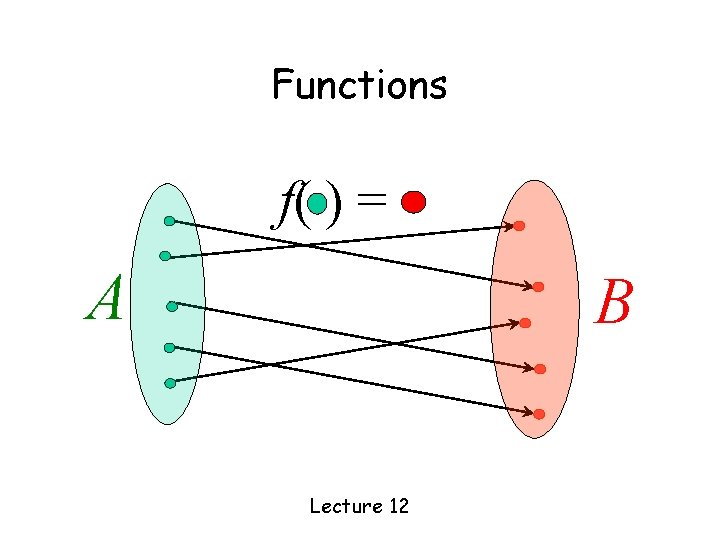Functions f( ) = A B Lecture 12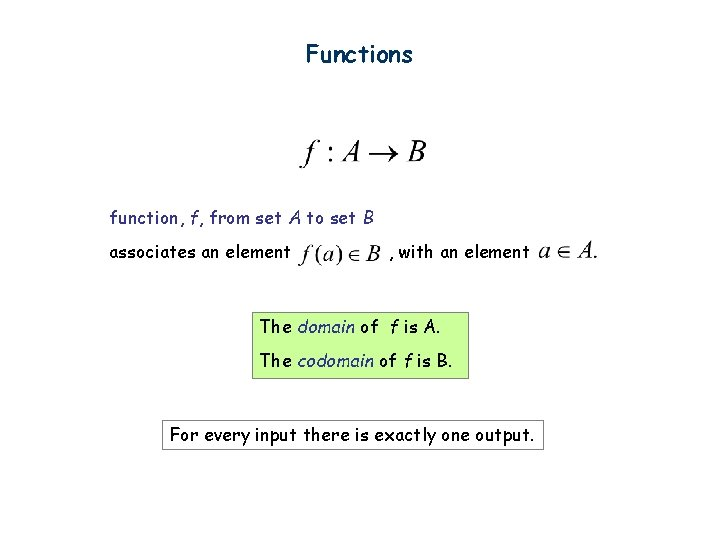Functions function, f, from set A to set B associates an element , with an element The domain of f is A. The codomain of f is B. For every input there is exactly one output.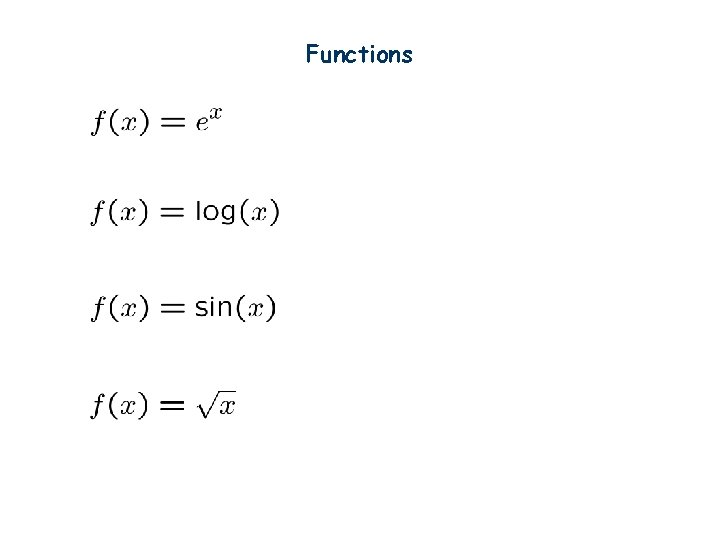FunctionsFunctions f(S) = |S| f(string) = length(string) f(student) = student-ID f(x) = is-prime(x)Injections (one-to-one) is an injection iff every element of B is f of at most 1 thing f( ) = A ≤ 1 arrow in B |A| ≤ |B|Surjections (Onto) is a surjection iff every element of B is f of something f( ) = 1 arrow in A B |A| ≥ |B|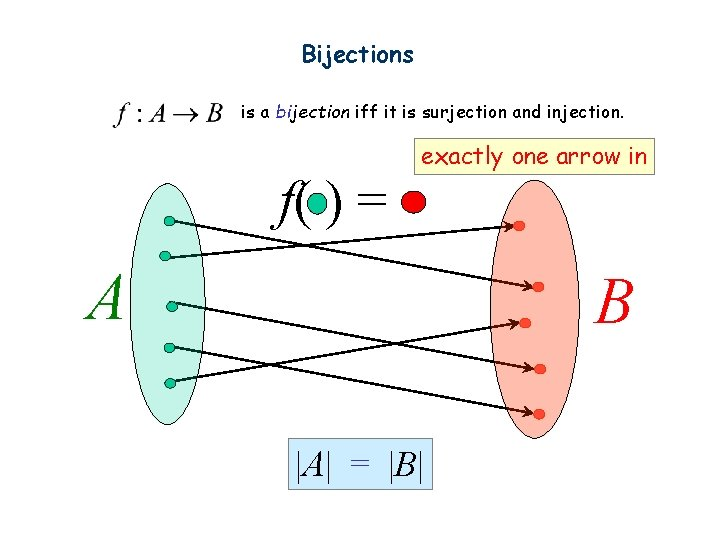Bijections is a bijection iff it is surjection and injection. f( ) = exactly one arrow in A B |A| = |B|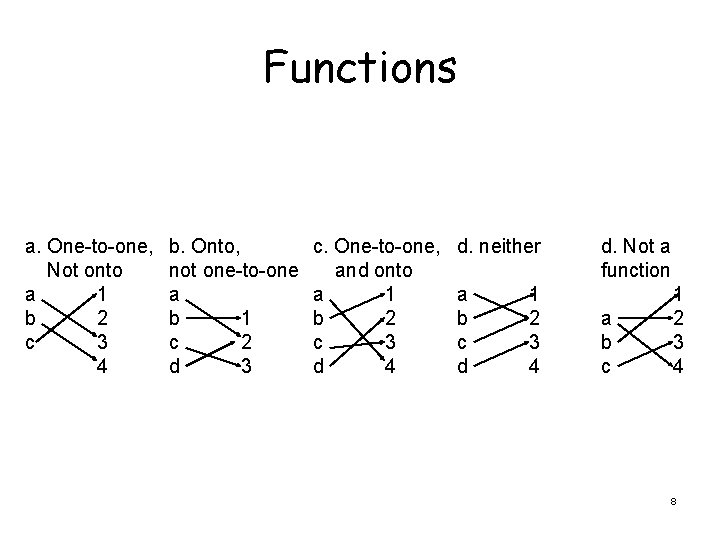Functions a. One-to-one, Not onto a 1 b 2 c 3 4 b. Onto, not one-to-one a b 1 c 2 d 3 c. One-to-one, and onto a 1 b 2 c 3 d 4 d. neither a b c d 1 2 3 4 d. Not a function a b c 1 2 3 4 8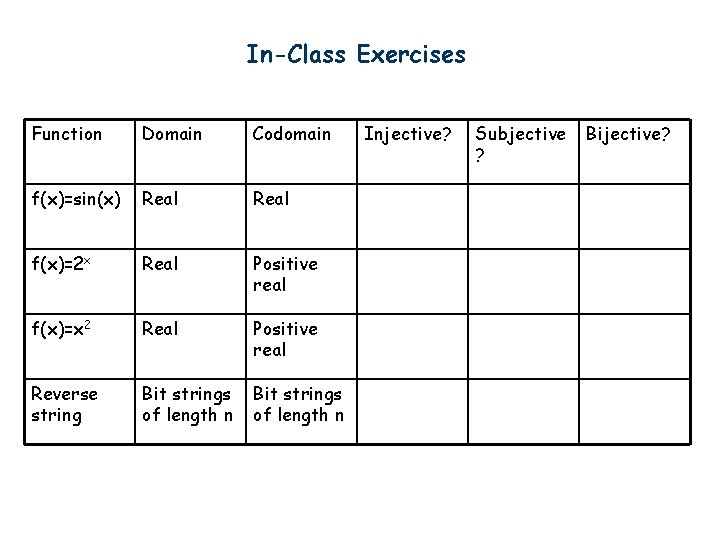In-Class Exercises Function Domain Codomain f(x)=sin(x) Real f(x)=2 x Real Positive real f(x)=x 2 Real Positive real Reverse string Bit strings of length n Injective? Subjective ? Bijective?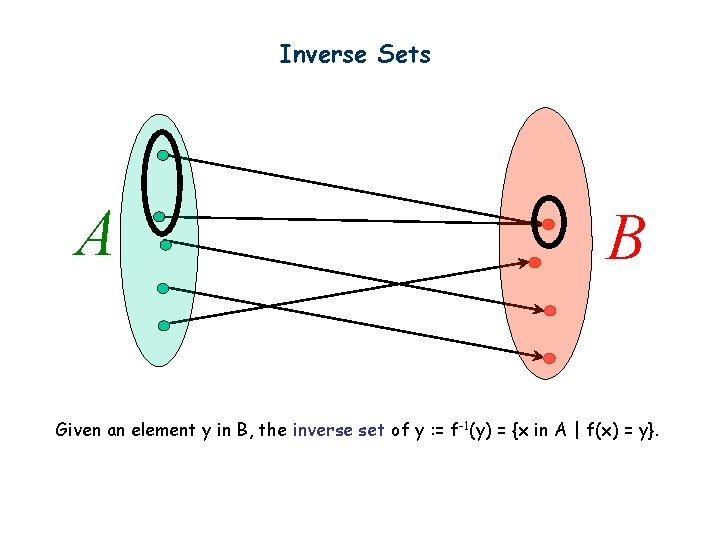Inverse Sets A B Given an element y in B, the inverse set of y : = f-1(y) = {x in A | f(x) = y}.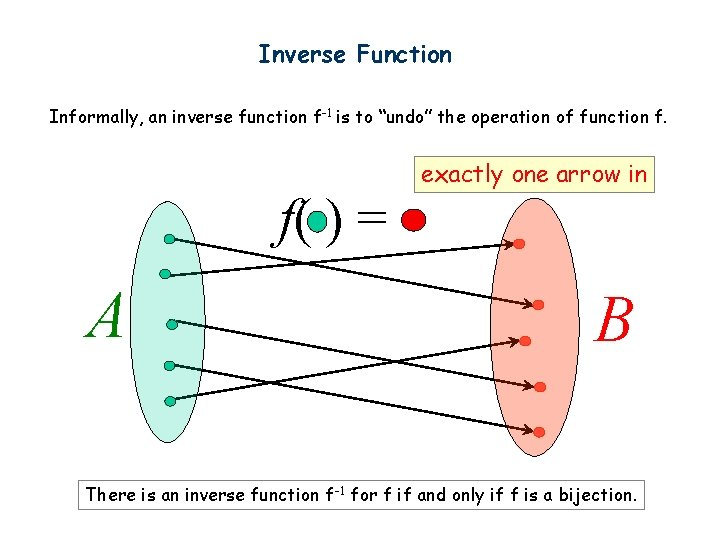Inverse Function Informally, an inverse function f-1 is to “undo” the operation of function f. f( ) = A exactly one arrow in B There is an inverse function f-1 for f if and only if f is a bijection.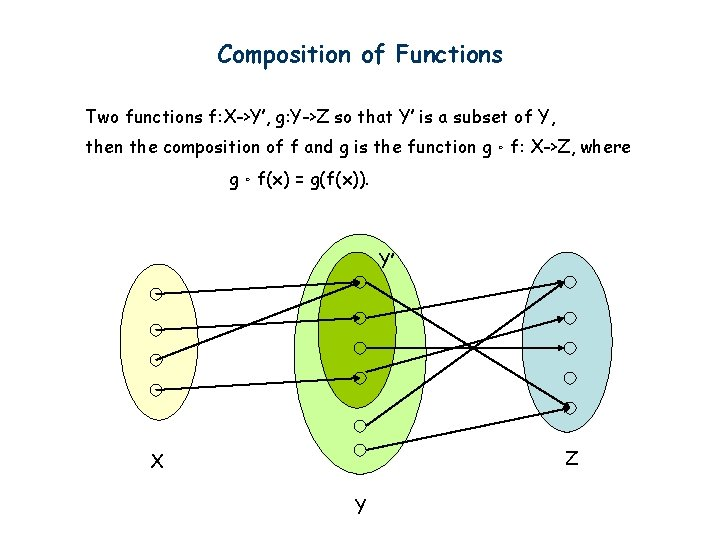Composition of Functions Two functions f: X->Y’, g: Y->Z so that Y’ is a subset of Y, then the composition of f and g is the function g。f: X->Z, where g。f(x) = g(f(x)). Y’ Z X YIn-Class Exercises Function f Function g f: X->Y f subjective g: Y->Z g injective f: X->Y f subjective g: Y->Z g subjective f: X->Y f injective g: Y->Z g subjective f: X->Y f bijective g: Y->Z g bijective f: X->Y F-1: Y->X Injective? Subjective? Bijective?